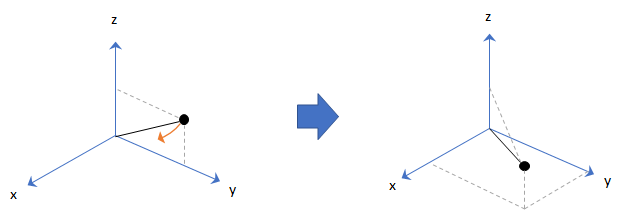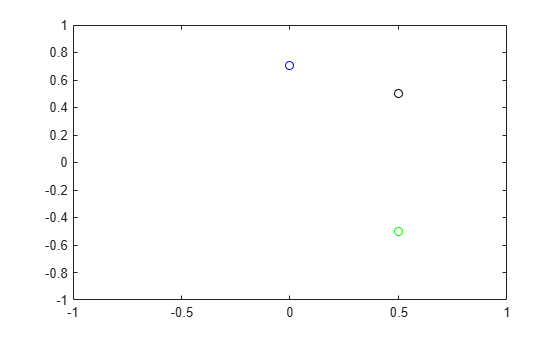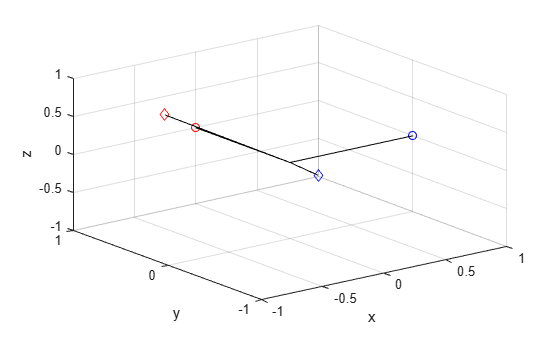# rotatepoint

Quaternion point rotation

## Syntax

``rotationResult = rotatepoint(quat,cartesianPoints)``

## Description

example

````rotationResult = rotatepoint(quat,cartesianPoints)` rotates the Cartesian points using the quaternion, `quat`. The elements of the quaternion are normalized before use in the rotation.```

## Examples

collapse all

Define a point in three dimensions. The coordinates of a point are always specified in order x, y, z. For convenient visualization, define the point on the x-y plane.

```x = 0.5; y = 0.5; z = 0; plot(x,y,'ko') hold on axis([-1 1 -1 1])```Create a quaternion vector specifying two separate rotations, one to rotate the point 45 and another to rotate the point -90 degrees about the z-axis. Use `rotatepoint` to perform the rotation.

```quat = quaternion([0,0,pi/4; ... 0,0,-pi/2],'euler','XYZ','point'); rotatedPoint = rotatepoint(quat,[x,y,z])```
```rotatedPoint = 2×3 -0.0000 0.7071 0 0.5000 -0.5000 0 ```

Plot the rotated points.

```plot(rotatedPoint(1,1),rotatedPoint(1,2),'bo') plot(rotatedPoint(2,1),rotatedPoint(2,2),'go')```Define two points in three-dimensional space. Define a quaternion to rotate the point by first rotating about the z-axis 30 degrees and then about the new y-axis 45 degrees.

```a = [1,0,0]; b = [0,1,0]; quat = quaternion([30,45,0],'eulerd','ZYX','point');```

Use `rotatepoint` to rotate both points using the quaternion rotation operator. Display the result.

`rP = rotatepoint(quat,[a;b])`
```rP = 2×3 0.6124 0.5000 -0.6124 -0.3536 0.8660 0.3536 ```

Visualize the original orientation and the rotated orientation of the points. Draw lines from the origin to each of the points for visualization purposes.

```plot3(a(1),a(2),a(3),'bo'); hold on grid on axis([-1 1 -1 1 -1 1]) xlabel('x') ylabel('y') zlabel('z') plot3(b(1),b(2),b(3),'ro'); plot3(rP(1,1),rP(1,2),rP(1,3),'bd') plot3(rP(2,1),rP(2,2),rP(2,3),'rd') plot3([0;rP(1,1)],[0;rP(1,2)],[0;rP(1,3)],'k') plot3([0;rP(2,1)],[0;rP(2,2)],[0;rP(2,3)],'k') plot3([0;a(1)],[0;a(2)],[0;a(3)],'k') plot3([0;b(1)],[0;b(2)],[0;b(3)],'k')```## Input Arguments

collapse all

Quaternion that defines rotation, specified as a scalar quaternion, row vector of quaternions, or column vector of quaternions.

Data Types: `quaternion`

Three-dimensional Cartesian points, specified as a 1-by-3 vector or N-by-3 matrix.

Data Types: `single` | `double`

## Output Arguments

collapse all

Rotated Cartesian points defined using the quaternion rotation, returned as a vector or matrix the same size as `cartesianPoints`.

Data Types: `single` | `double`

## Algorithms

Quaternion point rotation rotates a point specified in R3 according to a specified quaternion:

`${L}_{q}\left(u\right)=qu{q}^{*}$`

where q is the quaternion, * represents conjugation, and u is the point to rotate, specified as a quaternion.

For convenience, the `rotatepoint` function takes in a point in R3 and returns a point in R3. Given a function call with some arbitrary quaternion, q = a + bi + cj + dk, and arbitrary coordinate, [x,y,z], for example,

`rereferencedPoint = rotatepoint(q,[x,y,z])`
the `rotatepoint` function performs the following operations:

1. Converts point [x,y,z] to a quaternion:

`${u}_{q}=0+xi+yj+zk$`
2. Normalizes the quaternion, q:

`${q}_{n}=\frac{q}{\sqrt{{a}^{2}+{b}^{2}+{c}^{2}+{d}^{2}}}$`
3. Applies the rotation:

`${v}_{q}=q{u}_{q}{q}^{*}$`
4. Converts the quaternion output, vq, back to R3

## Extended Capabilities

### Objects

Introduced in R2020a